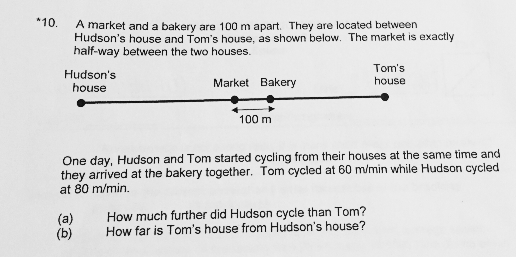# QuestionAnyone help me with attached question

Thanks

Hudson to market = Tom to market = D

Hudson cycled (D + 100)

Tom cycled (D – 100)

(D + 100) – (D – 100) = 200 m

(a) Hudson cycled 200m more

They both cycled for t mins.

80t – 60t = 200

t = 200 ÷ 20 = 10 mins

80t + 60t = 800 + 600 = 1400 m

(b) The houses are 1400m apart.

0 Replies 2 Likes ✔Accepted Answer
(a)
distance Tom travelled ——- 1u – 100
distance Hudson travelled ——- 1u + 100
1u + 100 – (1u – 100) = 200

(b)
(80 x time taken) – (60 x time taken)——- 200
time taken ——- 200/(80 – 60) = 10
80 x 10 + 60 x 10 = 1400

Ans : (a) 200 m; (b) 1400 m.
0 Replies 1 Like